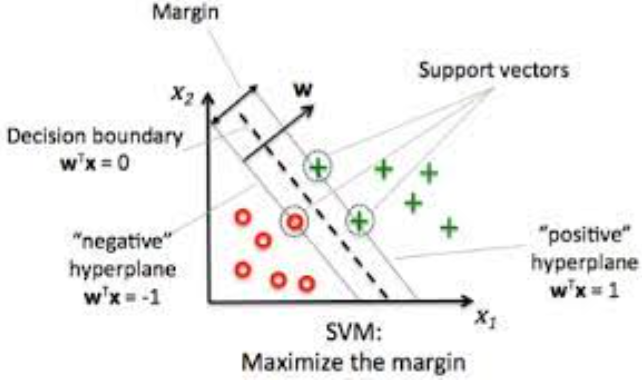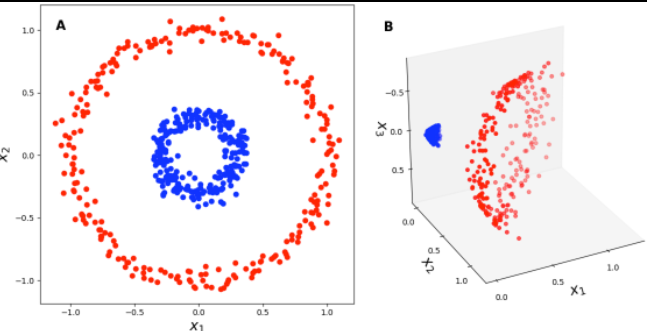# Support Vector Machines

dougieduk·2022년 4월 4일
0

# Basic Concept

• supervised machine learning models with associated learning algorithms that analyze data and recognize patterns, used for classification and regression analysis
• non-probabilistic binary linear classifier
• a representation of the examples as points in space, mapped so that the examples of the separate categories are divided by a clear gap that is as wide as possible
- new examples are mapped into the same space
• Objective : choose the line (hyperplane) which has the largest margin• Kernel tricks can be used for nonlinear data